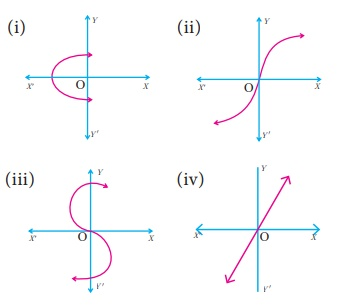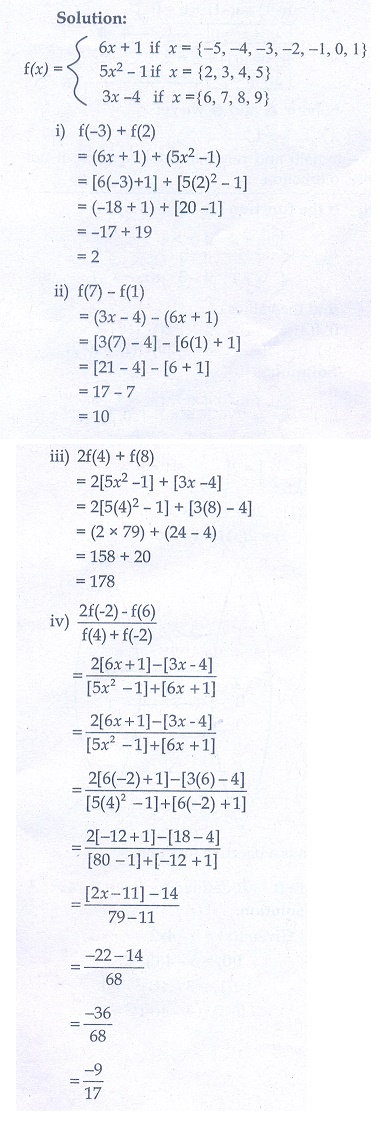Home | | Maths 10th Std | Exercise 1.4: Types of Functions

# Exercise 1.4: Types of Functions

Maths Book back answers and solution for Exercise questions - Mathematics : Relation and Function: Types of Functions: Exercise Problem Questions with Answer

Exercise 1.4

1. Determine whether the graph given below represent functions. Give reason for your answers concerning each graph.2. Let → be a function defined by (x) =( x/2) − 1, where   A = {2, 4, 6,10,12} ,B = {0,1, 2, 4, 5, 9} . Represent f  by

(i) set of ordered pairs;

(ii) a table;

(iii) an arrow diagram;

(iv) a graph3. Represent the function  f  = {(1, 2),(2, 2),(3, 2),(4, 3),(5, 4)} through

(i) an arrow diagram

(ii) a table form

(iii) a graph4. Show that the function N → N defined by (x) = 2– 1 is one-one but not onto.5. Show that the function N → N defined by (m) = m2 + + 3 is one-one function.6. Let = {1, 2, 3, 4} and N Let f→ B be defined by (x) = x3 then,

(i) find the range of f

(ii) identify the type of function7. In each of the following cases state whether the function is bijective or not. Justify your answer .

(i) f R → R defined by (x) = 2+ 1)

(ii)  R → R defined by (x) = 3 – 4x28. Let = {−1,1} and  = {0, 2} . If the function  f→ B defined by (x) = ax is

an onto function? Find and b.9. If the function is defined by (x)=find the values of

(i) (3)

(ii) (0)

(iii) (−1  5)

(iv) (2) + (−2)10. A function : [−5, 9] → R is defined as follows:

(x) =Find

(i) (−3) + (2)

(ii) (7) - (1)

(iii) 2(4) + (8)

(iv)11. The distance an object travels under the influence of gravity in time seconds is given by S(t) = 1/2 gt2 + at where, (is the acceleration due to gravity), aare constants. Check if the function S(t) is one-one.12. The function ‘t’ which maps temperature in Celsius (C) into temperature in Fahrenheit (F) is defined by t() = where = 9/5 ( C ) + 32 . Find,

(i) t(0)

(ii) t(28)

(iii) t(-10)

(iv) the value of when t() = 212

(v) the temperature when the Celsius value is equal to the Farenheit value.1. (i) Not a function (ii) function (iii) Not a function (iv) function

2.(i) {(2,0),(4,1),(6,2),(10,4),(12,5)}6.(i) {1, 8, 27, 64} (ii) one-one and into function

7.(i) Bijective function (ii) Not bijective function 8. 1,1

9.(i) 5 (ii) 2 (iii) -2. 5 (iv) 1

10.(i) 2 (ii) 10 (iii) 178 (iv) -9/17

11. Yes 12.(i) 32°F (ii) 82. 4°F (iv) 24°C

(iv) 100°C (v) − 40°

Tags : Problem Questions with Answer, Solution | Mathematics , 10th Mathematics : UNIT 1 : Relation and Function
Study Material, Lecturing Notes, Assignment, Reference, Wiki description explanation, brief detail
10th Mathematics : UNIT 1 : Relation and Function : Exercise 1.4: Types of Functions | Problem Questions with Answer, Solution | Mathematics